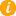# Pi## What Is Pi?

The number Pi is the ratio of a circle's circumference to its diameter, approximately equal to 3.14159...infinity. Whereas the circumference is the total distance around the outer edge of the circle and the diameter is the distance across the exact center of the circle. Most importantly, no mater the size of a circle, the division (ratio) of these two numbers will always be Pi 3.14159...infinity.

It has been represented by the Greek letter π though it is also sometimes spelled out as Pi (pai). Being an Irrational Number:
• Pi cannot be expressed exactly as a common fraction.
• Pi although fractions such as 22/7 and other rational numbers are commonly used to approximate π.
• Pi Its decimal representation never ends.
• Pi never settles into a permanent repeating pattern.